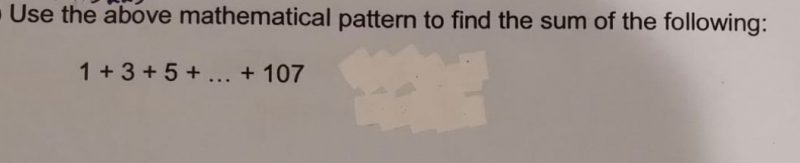# QuestionNotice that 1 +3 + 5 + …. + 103 + 105 + 107

can be paired off. 1 with 107,    3 with 105,     5 with 103

The sum of each pair is 108

Now, number of terms = (107 +1)/2 = 54

( notice that for 1+ 3 +5  , there are 3 terms,  (5+1)/2 =3 )

Number of pairs = number of pairs /2 = 27

The sum = 27(108)= 2916

0 Replies 2 Likes